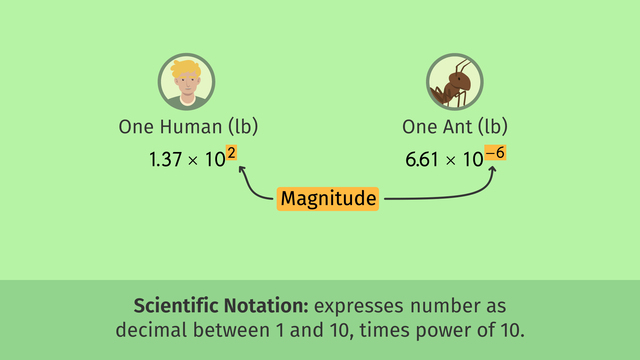# Multiplying and Dividing Numbers in Scientific NotationRating

Be the first to give a rating!

The authorsChris S.

## Basics on the topicMultiplying and Dividing Numbers in Scientific Notation

After this lesson you will be able to compare magnitudes using numbers in scientific notation.

The lesson begins with a review of how to express numbers in scientific notation and compare magnitude. This leads to a strategy for multiplying and dividing numbers in scientific notation, using the Laws of Exponents.

Learn how to multiply and divide numbers in scientific notation, by helping David compare the weights of ants to humans!

This video includes key concepts, notation, and vocabulary such as: scientific notation (a finite decimal between 1 and 10, times a power of 10); finite decimal (a terminating decimal); leading digit (the leftmost digit); and order of magnitude (the exponent of the power of 10).

Before watching this video, you should already be familiar with estimating and comparing numbers in scientific notation, and comparing magnitudes of numbers.

After watching this video, you will be prepared to work with larger or smaller numbers in decimal form and scientific notation.

Common Core Standard(s) in focus: 8.EE.3, 8.EE.4 A video intended for math students in the 8th grades Recommended for students who are 13-14 years old